#### You may also like### I'm Eight

Find a great variety of ways of asking questions which make 8.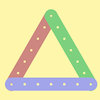### Let's Investigate Triangles

Vincent and Tara are making triangles with the class construction set. They have a pile of strips of different lengths. How many different triangles can they make?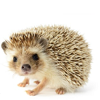### Noah

Noah saw 12 legs walk by into the Ark. How many creatures did he see?

# Even and Odd

## Even and Odd

This activity is best done as a whole class or in a large group.You will need to split up into four smaller groups: a black group, a blue group, a green group and a red group.

Each group will need the set of cards which has the same colour as their group name. Here are the sheets which will need printing off and cutting into separate cards: black cardsblue cards, green cardsred cards. In your group, can you pair up your cards so that each number card goes with a card which has a picture of that same number of coloured squares?

Use interlocking cubes, such as Multilink, to make the numbers on your cards in the same way as the pictures of coloured squares. For example, here is a block of Multilink which is $9$: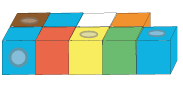Nine is an odd number. Could you tell this from looking at the diagram of the block of cubes? Eight is an even number. How would the shape of the block of eight cubes differ?

Choose two of your numbers made from Multilink. What happens when you add them by fitting the two Multilink 'blocks' together? What kind of number do you get? Is it odd or even?

Choose a different pair of numbers and add them in the same way. What do you get this time? Is the number odd or even?

In your group, try this in as many different ways as you can.

You could record what you are doing in some way.

When do you get an odd number?
When do you get an even number?

Can you explain why?

### Why do this problem?

This problem is designed to be worked on in groups so that lots of examples are generated. It offers opportunities for children to consolidate counting and addition, as well as giving them a way of 'seeing' odd and even numbers. The aim is for learners to be able to generalise the results of adding pairs of numbers together in terms of odds and evens.

### Possible approach

This activity is best done with the whole class, split into four smaller groups. One group will need a copy of these black cards, one will need the blue cards, one the green cards and the fourth group will need the red cards.

Ask learners to work in their groups to pair up their cards so that each number card goes with a card which has a picture of that same number of coloured squares. Then, supply each group with lots of Multilink cubes and challenge them to create a model of each number to look like the pictures of coloured squares. (You may need to demonstrate this as it is important that the cubes are paired, forming two 'rows'.) For example, here is the 'block' which represents $9$:Draw the whole class together and ask one group to choose two of their Multilink numbers. Hold up the two blocks and ask how they could add them together. How many cubes would we have altogether? Invite children to come up and demonstrate. They may come up with different ways to join the two blocks so spend some time exploring this. Which way makes it easier to count the number of cubes? Listen out for explanations which focus on counting in twos, so it might be easiest to try to make blocks which are based on two 'rows' of Multilink. For example, adding $9$ and $11$: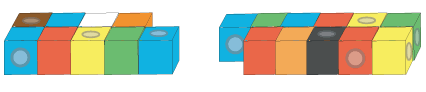Challenge each group to work together to add pairs of numbers together using the Multilink blocks they have made. You could invite them to record what they are doing, perhaps by shading squared paper to represent the blocks.

Bring the children together again and explain that you have joined two blocks together to make a larger block as they have been doing. Show them a block of, for example, $15$. What could the two smaller blocks have been? As children offer suggestions, draw representations of the numbers as squares, for example: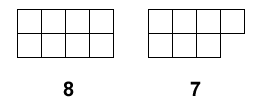Gather several examples on the board and ask children what they notice. What is the same about all the examples? Listen out for observations about one of the numbers having a square 'sticking out' and one of the numbers forming a rectangular block which doesn't have a 'sticking out' square. If the children do not already know the vocabulary of odds and evens, then introduce this to them. What kind of number is $15$? Encourage children to articulate that an odd number and an even number added together makes an odd number (or that an odd number can be made from one odd number and one even number added together).

Repeat this process with an even number, for example $12$. What two numbers could have been added together to make $12$? Gather examples on the board and encourage the generalisation in the same way: an odd number added to an odd number makes an even number, and an even number added to an even number makes an even number (or an even number can be made from two odd numbers added together or two even numbers added together).

### Key questions

How will you match the cards?
Which numbers have you tried adding together?
You could extend this challenge using a simplified version of Make $37$: If you are only allowed to use $1$s, $3$s and $5$s (but as many of each as you like), can you find all the ways to make $12$ using four numbers? Can you find all the ways to make $13$ using four numbers? This will allow you to assess whether they have fully understood the generalisation.